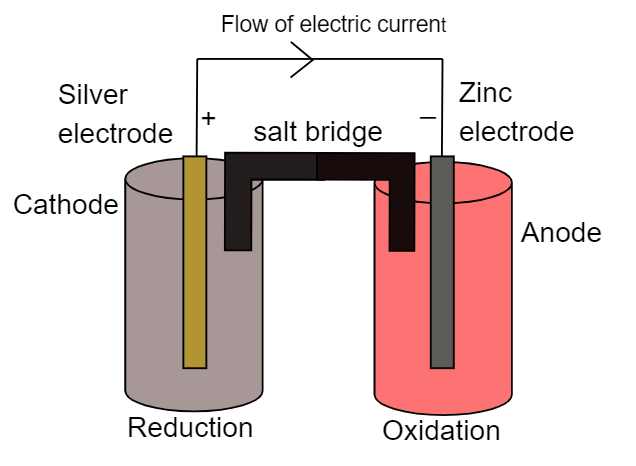# Draw the diagram of Galvanic cell which represents the following reaction: $Zn{\text{ }} + {\text{ }}2A{g^ + }{\text{ }} \to {\text{ }}Z{n^{2 + }} + {\text{ }}Ag$ Give an equation for the reaction taking place at each electrode.Verified
111.6k+ views
Hint: Galvanic cells are also known as electrochemical cells which consist of two electrodes namely cathode and electrode. At cathode reduction of metal takes place whereas at anode oxidation of metal takes place. Thus in galvanic cells redox reaction takes place consequently. Here zinc gets oxidized and silver gets reduced at their respective electrodes respectively.

Galvanic cell is one of the electrochemical chemicals which converts chemical energy into electrical energy with the help of redox reactions that take place at each electrode respectively. Here we have two electrodes namely cathode and electrode where reduction and oxidation reactions take place respectively. For the above cell representation we can write the half-cell reaction as:
At cathode:
Silver electrode acts as a cathode where reduction of silver ions takes place. It can be represented as:
$2A{g^ + }{\text{ + 2e }} \to {\text{ 2Ag}}$
At anode:
Zinc electrode acts as anode where oxidation of zinc takes place. It can be represented as:
$Zn{\text{ }} \to {\text{ Z}}{{\text{n}}^{2 + }}{\text{ }} + {\text{ }}2e$
Now the electron will flow from anode to cathode and thus the direction of conventional current will be vice-versa. Thus the chemical energy of the electrons is converted into electrical energy. It can be represented as:Note:
It must be noted that we have used a salt bridge which is used to maintain the electrical neutrality between the both beakers. We cannot replace the salt bridge with any other connecting medium. The direction of current is always opposite to that direction of flow of electrons. Thus the direction of flow of electric current will be from cathode to anode while the flow of electrons will be from anode to cathode.